کمیت: 0

مجموع: 0,00

# Grouping of solids

### Grouping of solids

This animation demonstrates various groups of solids through examples.

رياضى

کلیدواژه‌ها

grouping of solids, cylindrical objects, Rectangular prisms, prism, cuboid, cube, cylindrical, set, rectangular, solids, mathematics

### موارد مربوط#### Grouping of cuboids

This animation demonstrates various types of cuboids through everyday objects.#### محیط، مساحت، سطح و حجم

این انیمیشن فرمول های محاسبه محیط و مساحت شکل ها و همچنین مساحت و حجم احجام هندسی را نمایش می دهد.#### Building shapes (one colour)

Build 3D shapes from unit cubes with the help of several views.#### Conic sections

The conic section is a plane curve that is created when a right circular cone is intersected by a plane.#### Conic solids

This animation demonstrates various types of cones and pyramids.#### Cube

This animation demonstrates the components (vertices, edges, diagonals and faces) of the cube, one of the Platonic solids.#### Cuboid

A cuboid is a polyhedron with six rectangular faces.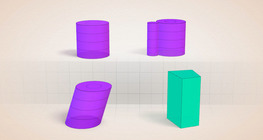#### Cylindrical solids

This animation demonstrates various types of cylindrical solids as well as their lateral surfaces.#### Grouping of solids 1

This animation demonstrates various groups of solids through examples.#### Grouping of solids 2

This animation demonstrates various groups of solids through examples.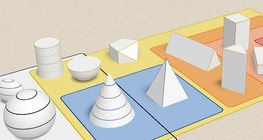#### Grouping of solids 3

This animation demonstrates various groups of solids through examples.#### Grouping of solids 4

This animation demonstrates various groups of solids through examples.#### Platonic solids

This animation demonstrates the five regular three-dimensional (or Platonic) solids, the best known of which is the cube.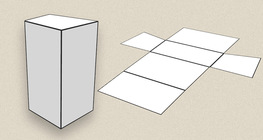#### Prisms

This animation demonstrates several types of prisms, from general to regular.#### Ratio of volumes of similar solids

This 3D scene explains the correlation between the ratio of similarity and the ratio of volume of geometric solids.#### Solids of revolution

Rotating a geometric shape around a line within its geometric plane as an axis results in a solid of revolution.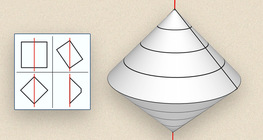#### Solids of revolution (exercise)

An exercise about the generation of solids of revolution.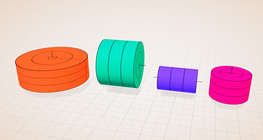#### Solids of revolution (rectangle)

Rotating a rectangle around its axes of symmetry or around its sides results in solids of revolution.#### Sphere

A sphere is the set of points which are all within the same distance from a given point in space.